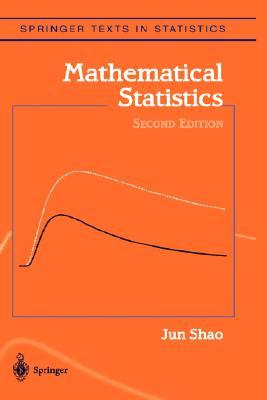## max vs. min

Posted in Books, Kids, Statistics with tags , , , , , , , , on March 26, 2022 by xi'anAnother intriguing question on X validated (about an exercise in Jun Shao’s book) that made me realise a basic fact about exponential distributions. When considering two Exponential random variables X and Y with possibly different parameters λ and μ,  Z⁺=max{X,Y} is dependent on the event X>Y while Z⁻=min{X,Y} is not (and distributed as an Exponential variate with parameter λ+μ.) Furthermore, Z⁺ is distributed from a signed mixture$\frac{\lambda+\mu}{\mu}\mathcal Exp(\lambda)-\frac{\lambda}{\mu}\mathcal Exp(\lambda+\mu)$

conditionally on the event X>Y, meaning that there is no sufficient statistic of fixed dimension when given a sample of n realisations of Z⁺’s along with the indicators of the events X>Y…. This may explain why there exists an unbiased estimator of λ⁻¹-μ⁻¹ in this case and (apparently) not when replacing Z⁺ by Z⁻. (Even though the exercise asks for the UMVUE.)

## extinction minus one

Posted in Books, Kids, pictures, R, Statistics, University life with tags , , , , , , , , , , , , , , , on March 14, 2022 by xi'an

The riddle from The Riddler of 19 Feb. is about the Bernoulli Galton-Watson process, where each individual in the population has one or zero descendant with equal probabilities: Starting with a large population os size N, what is the probability that the size of the population on the brink of extinction is equal to one? While it is easy to show that the probability the n-th generation is extinct is$\mathbb{P}(S_n=0) = 1 - \frac{1}{2^{nN}}$

I could not find a way to express the probability to hit one and resorted to brute force simulation, easily coded

for(t in 1:(T<-1e8)){N=Z=1e4
while(Z>1)Z=rbinom(1,Z,.5)
F=F+Z}
F/T


which produces an approximate probability of 0.7213 or 0.714. The impact of N is quickly vanishing, as expected when the probability to reach 1 in one generation is negligible…

However, when returning to Dauphine after a two-week absence, I presented the problem with my probabilist neighbour François Simenhaus, who immediately pointed out that this probability was more simply seen as the probability that the maximum of N independent geometric rv’s was achieved by a single one among the N. Searching later a reference for that probability, I came across the 1990 paper of Bruss and O’Cinneide, which shows that the probability of uniqueness of the maximum does not converge as N goes to infinity, but rather fluctuates around 0.72135 with logarithmic periodicity. It is only when N=2^n that the sequence converges to 0.721521… This probability actually writes down in closed form as$N\sum_{i=1}^\infty 2^{-i-1}(1-2^{-i})^{N-1}$

(which is obvious in retrospect!, albeit containing a typo in the original paper which is missing a ½ factor in equation (17)) and its asymptotic behaviour is not obvious either, as noted by the authors.

On the historical side, and in accordance with Stiegler’s law, the Galton-Watson process should have been called the Bienaymé process! (Bienaymé was a student of Laplace, who successively lost positions for his political idea, before eventually joining Académie des Sciences, and later founding the Société Mathématique de France.)

## more breaking sticks

Posted in Statistics with tags , , on July 19, 2021 by xi'an

A quick riddle from The Riddler that, when thinned to the actual maths problem, ends up asking for$\mathbb P(\max(U_1,U_2,U_3)=U_3)+\mathbb P(\min(U_1,U_2,U_3)=U_3)$

which is equal to 2/3…. Anticlimactic.

## new order

Posted in Books, Kids, R, Statistics with tags , , , , on February 5, 2021 by xi'anThe latest riddle from The Riddler was both straightforward: given four iid Normal variates, X¹,X²,X³,X⁴, what is the probability that X¹+X²<X³+X⁴ given that X¹<X³ ? The answer is ¾ and it actually does not depend on the distribution of the variates. The bonus question is however much harder: what is this probability when there are 2N iid Normal variates?I posted the question on math.stackexchange, then on X validated, but received no hint at a possible simplification of the probability. And then erased the questions. Given the shape of the domain where the bivariate Normal density is integrated, it sounds most likely there is no closed-form expression. (None was proposed by the Riddler.) The probability decreases roughly in N³ when computing this probability by simulation and fitting a regression.

> summary(lm(log(p)~log(r)))

Residuals:
Min        1Q    Median        3Q       Max
-0.013283 -0.010362 -0.000606  0.007835  0.039915

Coefficients:
Estimate Std. Error t value Pr(>|t|)
(Intercept) -0.111235   0.008577  -12.97 4.11e-13 ***
log(r)      -0.311361   0.003212  -96.94  < 2e-16 ***
---
Signif. codes:  0 ‘***’ 0.001 ‘**’ 0.01 ‘*’ 0.05 ‘.’ 0.1 ‘ ’ 1

Residual standard error: 0.01226 on 27 degrees of freedom
Multiple R-squared:  0.9971,	Adjusted R-squared:  0.997
F-statistic:  9397 on 1 and 27 DF,  p-value: < 2.2e-16


## where is .5?

Posted in Statistics with tags , , , , on September 10, 2020 by xi'an

A Riddler’s riddle on breaking the unit interval into 4 random bits (by which I understand picking 3 Uniform realisations and ordering them) and finding the length of the bit containing ½ (sparing you the chore of converting inches and feet into decimals). The result can be found by direct integration since the ordered Uniform variates are Beta’s, and so are their consecutive differences, leading to an average length of 15/32. Or by raw R simulation:

simz=t(apply(matrix(runif(3*1e5),ncol=3),1,sort))
mean((simz[,1]>.5)*simz[,1]+
(simz[,1]<.5)*(simz[,2]>.5)*(simz[,2]-simz[,1])+
(simz[,2]<.5)*(simz[,3]>.5)*(simz[,3]-simz[,2])+
(simz[,3]<.5)*(1-simz[,3]))


Which can be reproduced for other values than ½, showing that ½ is the value leading to the largest expected length. I wonder if there is a faster way to reach this nice 15/32.